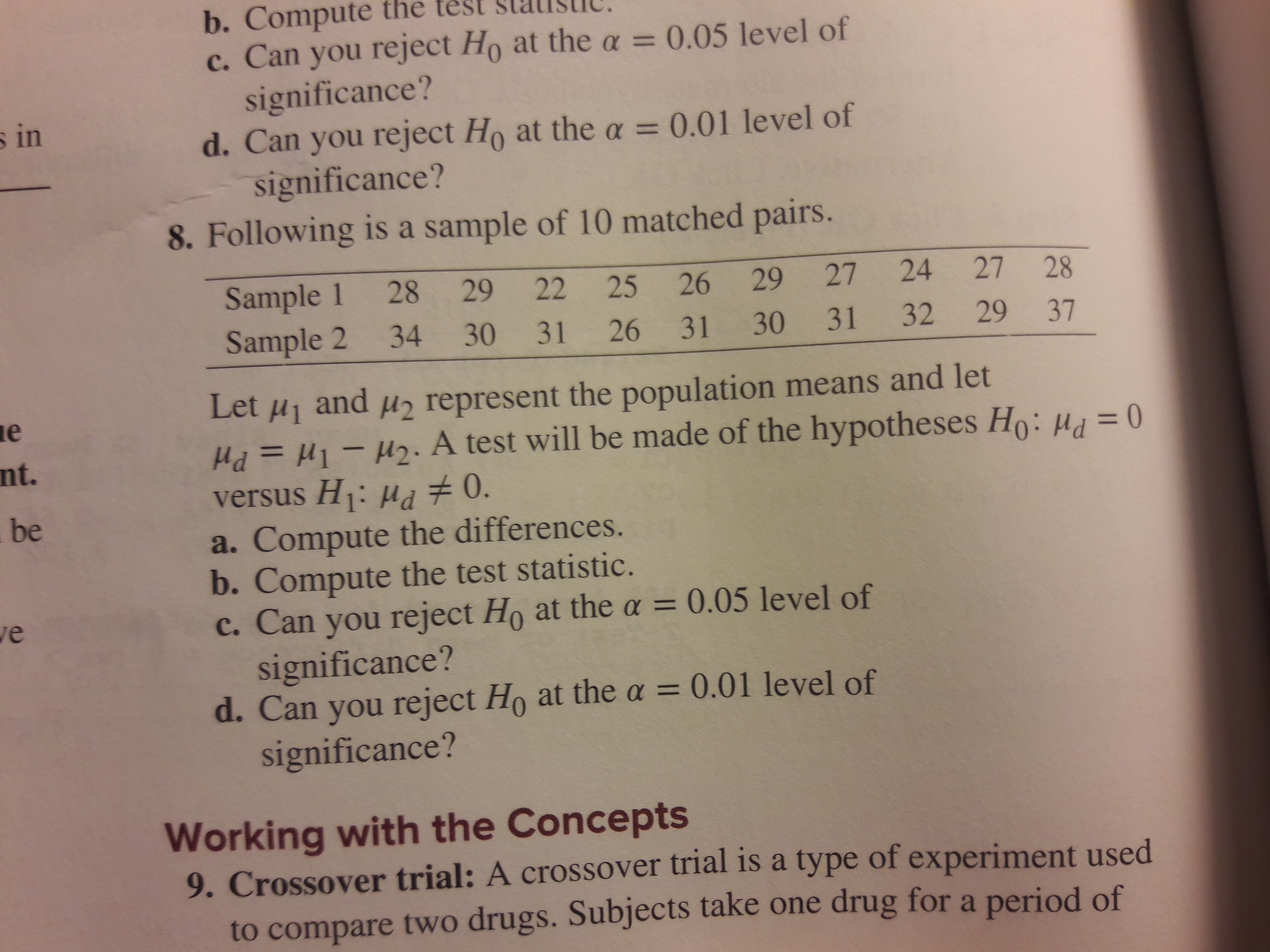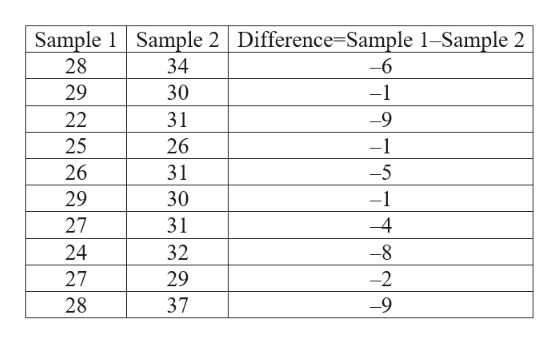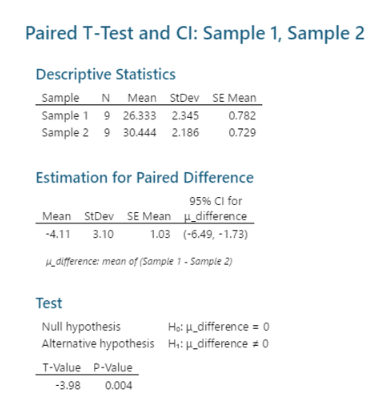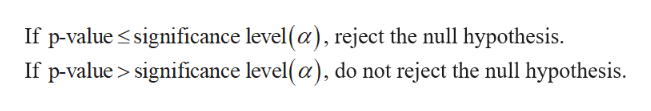# b. Compute the test st ic. Can you reject Ho at the a = 0.05 level ofsignificance?d. Can you reject Ho at thea = 0.01 level ofsignificance?8. Following is a sample of 10 matched pairs.s inSample 1 28 29Sample 2 34 3022 25262927 2427 2831 2631 30 31 322937Let H1andH represent the population means and letePd=11-am. A test will be made of the hypotheses Ho: H0versus H: ud 0.a. Compute the differences.b. Compute the test statistic.c. Can you reject Ho at the a = 0.05 level ofsignificance?d. Can you reject Ho at the a = 0.01 level ofsignificance?nt.beveWorking with the Concepts9. Crossover trial: A crossover trial is a type of experiment usedto compare two drugs. Subjects take one drug for a period of

Question
6 views

number 8 a, b, and chelp_outlineImage Transcriptioncloseb. Compute the test st i c. Can you reject Ho at the a = 0.05 level of significance? d. Can you reject Ho at thea = 0.01 level of significance? 8. Following is a sample of 10 matched pairs. s in Sample 1 28 29 Sample 2 34 30 22 25 26 29 27 24 27 28 31 26 31 30 31 32 29 37 Let H1 and H represent the population means and let e Pd=11-am. A test will be made of the hypotheses Ho: H0 versus H: ud 0. a. Compute the differences. b. Compute the test statistic. c. Can you reject Ho at the a = 0.05 level of significance? d. Can you reject Ho at the a = 0.01 level of significance? nt. be ve Working with the Concepts 9. Crossover trial: A crossover trial is a type of experiment used to compare two drugs. Subjects take one drug for a period of fullscreen
check_circle

Step 1

(a)

The difference is tabulated below:help_outlineImage TranscriptioncloseSample 1 Sample 2 Difference=Sample 1-Sample 2 28 34 -6 30 29 -1 22 31 -9 25 26 -1 31 -5 26 -1 29 30 27 31 -4 24 32 -8 27 29 -2 28 37 -9 fullscreen
Step 2

(b)

Step-by-step procedure to obtain the test statistic using MINITAB software:

• Choose Stat > Basic Statistics > Paired t test for mean.
• Choose Each sample in one column.
• In Sample 1, Select Sample 1.
• In Sample 2, Select Sample 2.
• Choose Options.
• In Confidence level, enter 95.
• In Alternative, select Not equal.
• Click OK in all the dialogue boxes.

Output obtained using MINITAB software is given below:help_outlineImage TranscriptionclosePaired T-Test and Cl: Sample 1, Sample 2 Descriptive Statistics Sample N Mean StDev SE Mean Sample 1 9 26.333 2.345 Sample 2 9 30.444 2.186 0.782 0.729 Estimation for Paired Difference 95% CI for Mean StDev SE Mean difference 1.03 (-6.49, -1.73) -4.11 3.10 dufference: mean of (Sample 1- Sample 2) Test Null hypothesis Alternative hypothesis H:difference 0 Ho: 4_difference = o T-Value P-Value -3.98 0.004 fullscreen
Step 3

From the output, the test statistics is –3.98 and the p-value is 0.004...help_outlineImage TranscriptioncloseIf p-value significance level(a), reject the null hypothesis. If p-value> significance level(a), do not reject the null hypothesis. fullscreen

### Want to see the full answer?

See Solution

#### Want to see this answer and more?

Solutions are written by subject experts who are available 24/7. Questions are typically answered within 1 hour.*

See Solution
*Response times may vary by subject and question.
Tagged in

### Statistics